# Forces

## Introduction

So far, we’ve just described how objects move, without much investigation into why.

Science has to be more than just descriptive though.*

This really gets at the notion of science as a predictor. We need to be able to understand what will happen, not just what has happened.

*Unless you’re in medicine, then you can just treat symptoms and ignore causesScience for many centuries was just concerned with observing the natural world.

Observations on the Storm of December 15, 1839Author(s): William C. Redfield Source: Transactions of the American Philosophical Society, New Series, Vol. 8 (1843), pp. 77- 82

#### We can do better.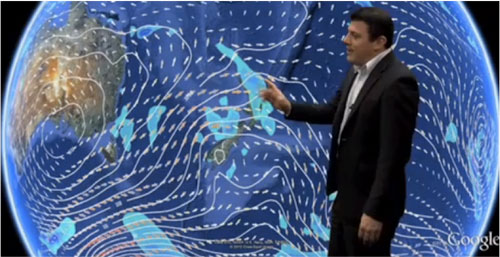(Vectors!)

#### A brief history

•Nicolaus Copernicus got himself into trouble by saying the earth revolved around the sun. [1473 - 1543]

•Tycho Brahe took a lot of very nice data points about the positions of planets. [1546 - 1601]

•Kepler, used Brahe’s data to figure out some basic rules which described planetary motion quite well. [1571-1630]

•Galileo had figured out $x \propto t^2$ for bodies under constant acceleration and that friction slows moving things down.[1564 – 1642]

#### Which brings us to 1680-1690• The English had recently taken over a quant village called "New Amsterdam" from the Dutch.
• The last dodo bird was killed.
• Halley's comet was observed (and someone predicted its return. Guess who?)
• J.S. Bach was born
• Choclate Milk was invented
• and...

#### The Principia was publishedIsaac Newton [1642-1727]

## The First LawEvery body continues in a state of rest, or of uniform motion in a right line, unless it is compelled to change that state by forces impressed upon it.If no net force acts on a body, its velocity won’t change.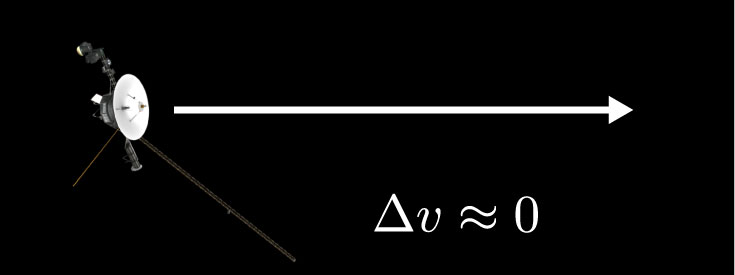The Voyager spacecraft

## Force

#### Classes of forces

1. Contact Forces: These forces involve physical contact between two bodies. Like pushing, pulling, rubbing, kicking, touching, etc.
2. Fields: A field can exert a force over a distance

#### How to describe a force

1. A force is like a push or a pull: The force is somehow responsible for the motion of another object
2. A force acts on an object: There was another object that moved. The force was applied to something.
3. A force requires an agent: There must be an 'agent' which acts on the object.
4. A force is a vector: A force has direction and magnitude, so it is certainly a vector quantity.

A ball is pushed briefly, and then rolls across the floor and comes to rest. It stopped moving because:

1. An invisible force was pushing against it.
2. Speed is proportional to force applied: No force means no speed.
3. Angels did it.
4. An object's natural state is at rest. Objects tend towards their natural states.

#### Multiple ForcesWhat about if we have multiple forces acting on an object.

Since we now know how to add vectors, we can handle this:

$$\sum \mathbf{F} = \mathbf{F}_\textrm{net} = \mathbf{F}_1 + \mathbf{F}_2 + \mathbf{F}_3 + \mathbf{F}_4 + \mathbf{F}_5 \ldots$$

Below are two forces, $\mathbf{F}_1$ and $\mathbf{F}_2$. We would like $\mathbf{F}_\textrm{net}$ to point straight north.

Which $\mathbf{F}_3$ could we add to make this happen?#### We can mix different forces: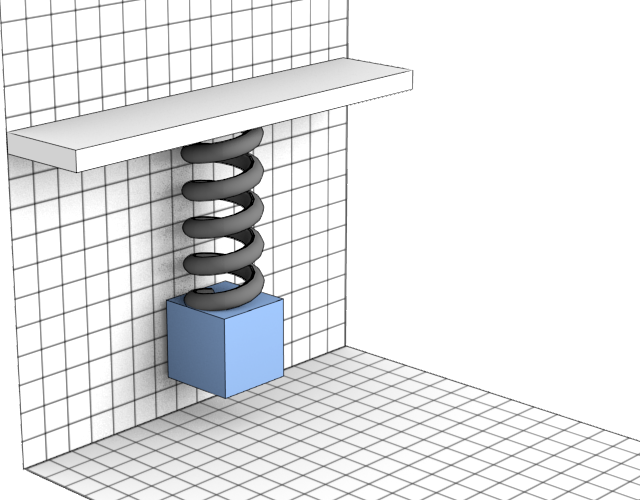Often, we'll have several different forces all acting on the same object.

For example, this box might have spring forces pulling it upwards, and weight pulling it downwards.

$$\sum \mathbf{F} = \mathbf{F}_\textrm{spring} + \mathbf{F}_\textrm{weight}$$

## Newton's second lawThe change in motion is ever proportional to the motive force impressed; and is made in the direction of the right line in which that force is impressed.The net force applied to a body produces a proportional acceleration in the same direction.

$$|\mathbf{F}| \propto |\mathbf{a}|$$ $$\mathbf{F} = m\frac{\Delta \mathbf{v}}{\Delta t} = m \mathbf{a}$$

The position of a particle along the x axis given by: $x(t) = 0.1 t^3 - 2 t^2 + 7 t$ (distances are in meters). Its motion is caused by mysterious forces which are due to magical spells cast on the particle by several druids. At what time do the forces of each druid cancel each other to create no net force? Where along the x axis does this happen? Is the particle moving at this time? If so in which direction and how fast?#### Same force, different masses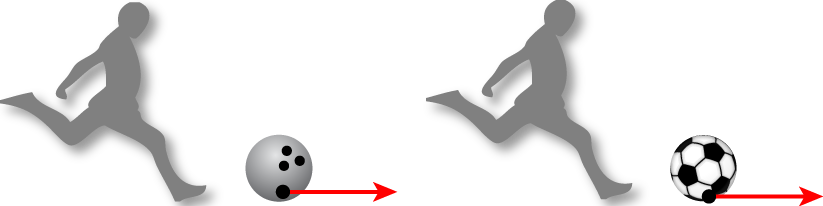Since $a = \frac{F}{m}$, if we apply the same force to objects of different mass, we can see that the one with the larger mass will experience less acceleration.

For the bowling ball: $$a_\textrm{bowling ball} = \frac{F_\textrm{kick}}{m_\textrm{bowling ball}}$$

For the soccer ball: $$a_\textrm{soccer ball} = \frac{F_\textrm{kick}}{m_\textrm{soccer ball}}$$

When a rubber band is stretched to pull on a 1.0 kg block with a constant force, the acceleration of the block is measured to be 3.0 m/s$^2$. When a block of unknown mass is pulled with the same rubber band, using the same force, its acceleration is 5.0 m/s$^2$. What is the mass of the unknown block?

### Free body diagrams

One of our conceptual models we'll use frequently involves the creation of a free body diagram.

As you sit quietly in your chair contemplating physics, the net force acting on you is:

1. Up (+y)
2. Zero
3. Down (-y)
5. Depends on the angle of the chair

When you're standing on the subway moving at a constant speed in the same forward (+x) direction, the net force on you is:

1. Up (+y)
2. Zero
3. Down (-y)
4. Forward (+x)
5. Backward (-x)

#### Equilibrium

When, after adding together all the forces acting on an object, we find that the sum equals zero, then we'll say that the object, or system, is in equilibrium.

When the net force is equal to zero:

1. The acceleration is equal to zero
2. The velocity is constant

Equilibrium occurs when the net force is equal to zero.

1. The object, if at rest, will remain at rest
2. If the object is moving, it will continue to move at a constant velocity

Re-write the first law using math:

1. $\mbox{if: }[\mathbf{F}_\textrm{applied} = 0] \mbox{ then } \left[ \frac{\Delta x}{\Delta t} = 0\right]$
2. $\mbox{if: } \left[ \sum \mathbf{F} = 0 \right] \mbox{ then } \left[ \frac{\Delta v}{\Delta t} = 0\right]$
3. $\mbox{if: } \left[ \mathbf{F_x} = \mathbf{F_y}\right] \mbox{ then } \left[ \mathbf{a_x} = \mathbf{a_y}\right]$
4. $\mbox{if: }\left[\mathbf{F}_\textrm{net} = 0 \right] \mbox{ then } \left[ v = 0 \right]$

## A Catalog of Forces

#### TensionsTension force is a contact force exerted by a rope or string as it pulls on an object.

The direction is always in the direction of the pulling.

Here, we usually assume the rope is massless.

#### Gravity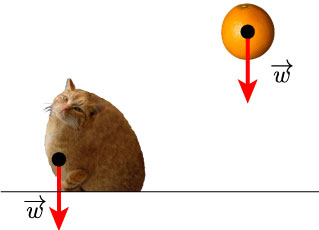Gravity exerts a force on all massive objects. We call this force weight.

#### The Normal Force

The normal is a term from geometry meaning perpendicular. We call the force exerted by a surface on an object that is pressing against the surface.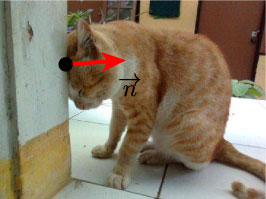#### Friction

When an object slides, or attempts to slide over a surface, it experiences a resistance to movement, due to bonding forces between it and surface.

This resistance is frictional force f, directed opposite to the (attempted) motion.

Two kinds: kinetic ($f_k$) and static ($f_s$)#### Spring or Restoring Forces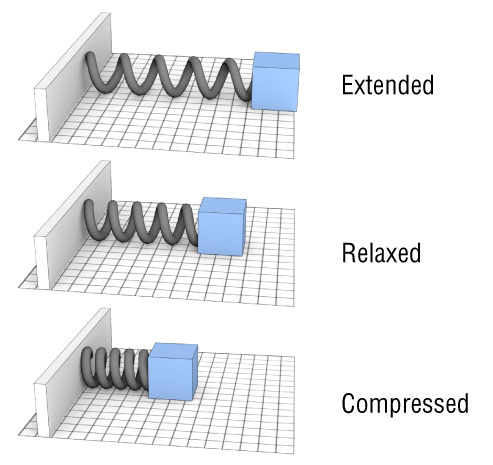#### Spring math

The force provided by a spring is proportional to its displacement. $$F_\textrm{spring} = k \Delta x.$$

And, since force is a vector, we can write $$\mathbf{F} = -k \Delta \mathbf{x}$$

$\Delta x$ is our standard displacement and $k$ is a constant called the spring constant. It tells us how stiff the spring is.#### Drag

Another resistive force is drag. (Also called air resistance - what we’ve been ignoring)

Keep on ignoring it unless specifically told otherwise.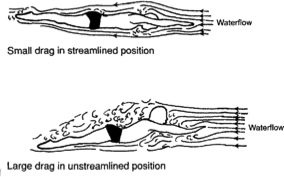## The Third Law“To every action there is always opposed an equal reaction: or the mutual actions of two bodies upon each other are always equal, and directed to contrary parts.”To every action there is always an equal and opposite reaction.

### Action/reaction pairs

$$\mathbf{F}_\textrm{box on hand} = - \mathbf{F}_\textrm{hand on box}$$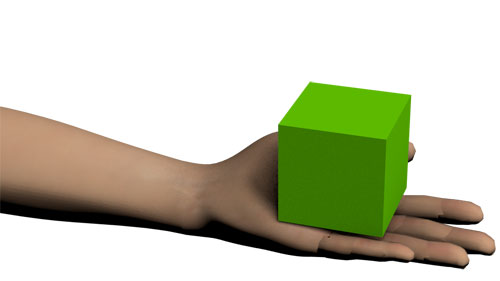The bat on ball force gets all the credit. But, we mustn't neglect the force of the ball on the bat. That is really the essence of the third law.Tactic: Identify the pairs:

The two forces in an action-reaction pair must act on different objects

The two forces in an action-reaction pair point in opposite directions and are equal in magnitude.A small car is pushing a large truck that has a dead battery. The mass of the truck is much greater than the mass of the car. Which of the following statements is true?

1. The car exerts a force on the truck, but the truck doesn't exert a force on the car.
2. The car exerts a larger force on the truck than the truck exerts on the car.
3. The car exerts the same amount of force on the truck as the truck exerts on the car.
4. The truck exerts a larger force on the car than the car exerts on the truck.
5. The truck exerts a force on the car, but the car doesn't exert a force on the truck.

How can it be moving if the forces on each thing are the same but pointed in the opposite direction? Shouldn't the forces cancel out?

#### In words:

1. No change in velocity if there is no net force acting.
2. A force will cause a proportional acceleration inversely proportional to the mass.
3. Forces come in equal and opposite pairs

#### In symbols:

1. $\Delta v = 0$ if $F_\textrm{net} = 0$
2. $\mathbf{F} = m\mathbf{a}$
3. $F_{AB} = -F_{BA}$

#### A minor qualification

Newton's laws are only applicable in this form if the system we are observing is not accelerating.

Another way of saying this: We must be in an inertial reference frame.

In a car crash, a car stops in 0.10 s from a speed of 14 m/s. The driver has a mass of 70kg. What is the force applied to the driver by the seat belt during the collision?Find the $a_x$ and $a_y$ for the object shown here. Its mass is 2.0 kg.

A particle has two forces acting on it. Force A points in the Y direction and has a magnitude $A$. Force B points in the X direction and has a magnitude of $B$. What is the magnitude of the total force acting on the particle?

1. $F_\textrm{net} = A + B$
2. $F_\textrm{net} = A - B$
3. $F_\textrm{net} = \sqrt{A^2 + B^2}$
4. $F_\textrm{net} = A^2 + B^2$

## Gravity

Gravity is an attractive force between masses.Its magnitude can be calculated by the following expression:

$$\bbox[15px,border:4px solid red]{F_\textrm{gravity} = G\frac{m_1 m_2}{r^2}}$$

$G$ is a constant and equals $6.674 \times 10^{-11}\;\rm N\cdot m^2/kg^2$

#### Mass vs. Weight

Mass is an inherent property of an object:

1. Mass is a scalar quantity, with SI units of kg
2. Mass is independent of the object’s surroundings
3. It is determined by the physical make up of the object.

Weight is equal to the magnitude of the gravitational force exerted on the object

1. An object’s weight can be zero far from any gravitating body, but mass will not change
2. The same object can have different weights depending on where it is located.

(In English, we use these interchangeably -- I'll try to be careful)

Two objects that may be considered point masses are initially separated by a distance d. The separation distance is then decreased to d/3. How does the gravitational force between these two objects change as a result of the decrease?

1. The force will not change since it is only dependent on the masses of the objects.
2. The force will be nine times larger than the initial value.
3. The force will be three times larger than the initial value.
4. The force will be one third of the initial value.
5. The force will be one ninth of the initial value.
Find the gravitational force between two people sitting next to each other in this room.
Find the gravitational force between the earth and a person.

#### Weight

The weight of an object on or above the earth is the gravitational force that the earth exerts on the object. The weight always acts downward, toward the center of the earth.

$$W = G\frac{M_E m }{r^2}$$

Here:
$G$, is the gravitational constant,
$M_E$ is the mass of the earth, $5.98 \times 10^{24}$ kg,
$m$ is the mass of the object,
and $r$ is the distance between the center of the earth and the object.

What are the units of weight?

#### Mass and Weight

We now have two equations for weight:

$$W = G\frac{M_E m }{r^2} = \left(G\frac{M_E }{r^2}\right) m$$
$$W = m g$$

An astronaut's weight on earth is 800N. What is his weight on Mars, where the acceleration due to gravity there is 3.76 m/s$^2$?

The international space station is about 250 miles above the surface of the earth. (The radius of the earth is 4000 miles) The force of gravity there is roughly what percent as strong as on the earth's surface?

1. 99%
2. 90%
3. 10%
4. 1%
5. 0.01%

A particle is at rest at the origin...

## The Normal force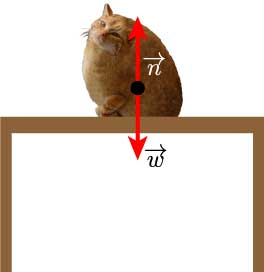Here we have a cat on a table. There are two forces acting on the kitty: the weight (gravitational force) between the earth and the cat, and the normal force of the table on the cat.

The magnitude of the normal force is equal to the weight force, and pointed in the opposite direction. $$\overrightarrow{W} = - \overrightarrow{n}$$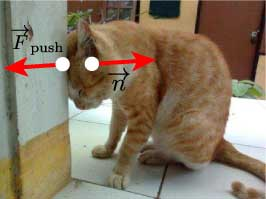This kitty is exerting a horizontal pushing force against the wall, and feels the normal force from the wall as a result.Here's a regular box on a regular table. The normal force is equal and opposite to the force due to gravity.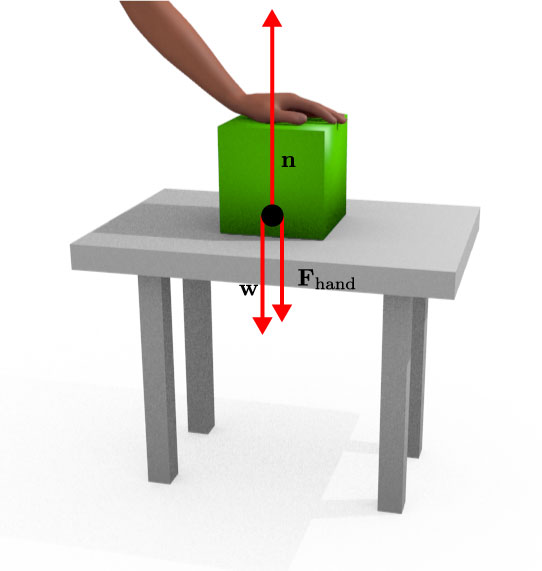Now a hand applies a downward force to the box. The normal force increases to match the combined $\mathbf{F}_\textrm{hand}$ and $\mathbf{w}$.

$$\mathbf{n} = -(\mathbf{w}+\mathbf{F}_\textrm{hand})$$Here's a regular box on a regular table. The normal force is equal and opposite to the force due to gravity.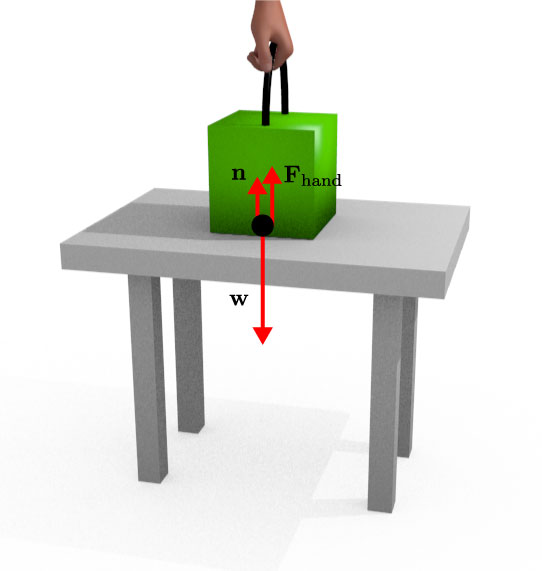Likewise, if our hand force pulls the block up instead, this force will cause a decrease in the normal force

$$\mathbf{n} = -(\mathbf{w}-|\mathbf{F}_\textrm{hand}|)$$What does this thing measure (no matter how I use it)?

1. Mass
2. Force due to gravity
3. Normal force applied
4. Acceleration

Vector $\mathbf{R}$ points straight down. What are the components of $\mathbf{R}$ parallel and perpendicular to the ramp?Draw a free body diagram for this box as it slides down this frictionless ramp. What is the magnitude of the normal force on the box? What is the acceleration of the box? Plot it as a function of time.For what angle of $\theta$ will the component of gravity acting perpendicular to the ramp surface be equal to one half the weight of the cat?

1. $\theta = 0^\circ$
2. $\theta = 30^\circ$
3. $\theta = 45^\circ$
4. $\theta = 60^\circ$
5. $\theta = 90^\circ$

If this 2kg box is moving towards the ramp on this frictionless surface, at 4 m/s:

(a) how long, starting from the position shown in the drawing, will it take to reach its maximum height?

(b) What is the height above the ground, $h$, where the block stops?I'm standing on a scale in an elevator. Nothing is moving. How many forces are acting on me?

1. 0
2. 1
3. 2
4. 3
5. 4Which would be a good free body diagram for me this situation (i.e. in an elevator and not moving)?Now, the elevator is accelerating up. How many forces are acting on me?

1. 0
2. 1
3. 2
4. 3
5. 4Which would be a good free body diagram for this situation (i.e. in an elevator and accelerating up)?#### True and Apparent Weight

Our formula for calculating the weight of an object relies on the assumption that the object is not accelerating towards or away from the earth: $$w = mg$$

However, if the object being weighed is accelerating in the $y$ axis, then we must account for that: $$n_\textrm{apparent}= mg + ma$$

This is what happens if you try to use a scale while the elevator is accelerating up or down.

Here is the velocity vs. time graph for an elevator. A person with mass $m$ is in the elevator standing on a scale.A 100 kg donkey rides in an elevator. Its velocity as a function of time is given by the following graph. What is the apparent weight of the donkey at $t = 1s, t = 5s$, and $t = 9s$?You let an apple fall from rest starting at a position...

## Tension Force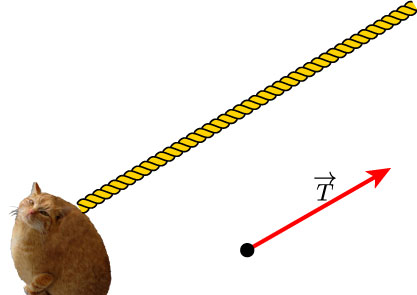Ropes offer us a way to move objects. They do this by applying a tension force.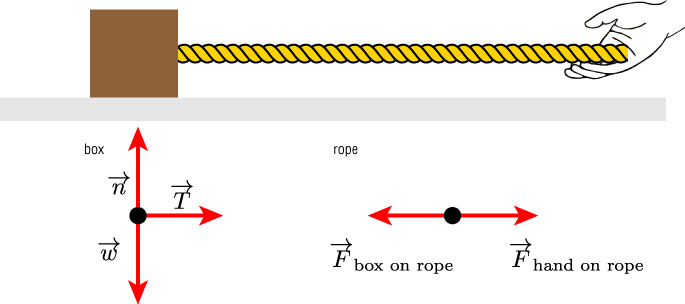Find an expression for the tension in the rope, based on the relevant parameters.

A box is resting on the ground, and is also partly suspended by a rope...

### Pulleys - what do they do?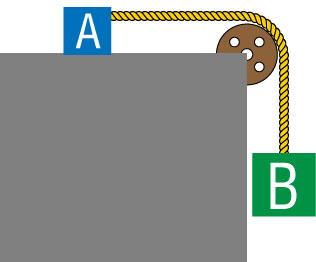They redirect a force: The weight of Mass B is usually directed in the -y direction. However, by using a pulley, we can use the rope to accelerate Block A in the x direction.

Again: some assumptions:

1. The pulleys we use are massless, just like the ropes.
2. A pulley is also frictionless on its axle.
3. Thus, none of the forces of a problem are ‘used’ to turn the pulley.

#### Free body diagram of the two objects.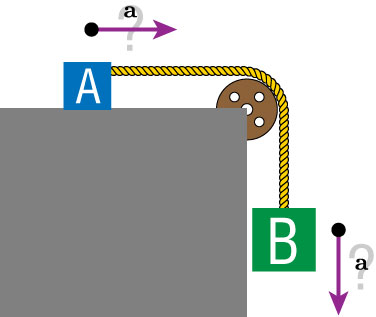The two boxes are connected by the rope. Compare the accelerations of masses A and B. (B is three times heavier than A)

1. Box A has a larger acceleration
2. Box B has a larger acceleration
3. Their accelerations are the same
4. Not enough information to determine

Find (a) the tension in the rope, and (b) the normal force acting on the blue box.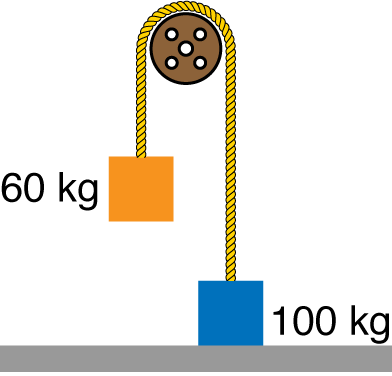If box B is accelerating down, what can we say about the tension acting on box B?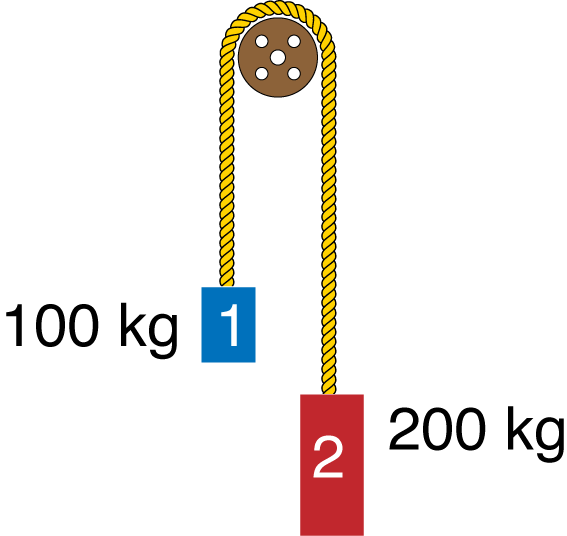Mass 2 is allowed to fall. It is connected via the rope and pulley to mass 1. What is the acceleration of mass 1?

#### Equilibrium Applications

Equilibrium for an object or system of interacting objects occurs when there is no acceleration of any of the objects.

$a_y = 0$, and $a_x = 0$

This implies the net forces in both directions are all zero as well:

$\sum F_x = 0$ and $\sum F_y = 0$

This does not imply that the velocity of the object(s) has to be zero.What is the angle $\theta$ in this diagram? The mass and the pulleys are all stationary.

#### Non-equilibrium Applications

In the case that there is an accelerating object, then we know the net force in that direction is not zero Newtons.

Instead of setting the net force equal to zero, it will equal the acceleration in that direction:

$$\sum F_x = F_{\textrm{net}_x} = m a_x$$

$$\sum F_y = F_{\textrm{net}_y} = m a_y$$

Find the acceleration of the 2 kg block.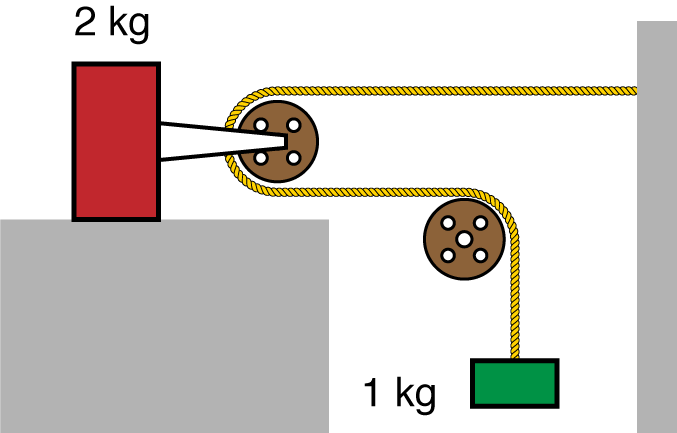A skier slides down a slope of 10 degrees. A wind blows along the direction of the slope. Let's say the skier has a mass of 40 kg. Find the acceleration of the skier as a function of the wind force. Sketch a plot of this function.

An elevator cab and its load have a combined mass of 1600 kg. Find the tension in the supporting cable when the cab, originally moving downward at 12 m/s, is brought to rest with constant acceleration in a distance of 42 m.

A box of mass $m$ is released from rest on a frictionless ramp as shown...

## Friction

To really understand friction, we would have to account for atomic scale forces and interactions. That’s hard!
Instead, we’ll make a model of the way friction forces work.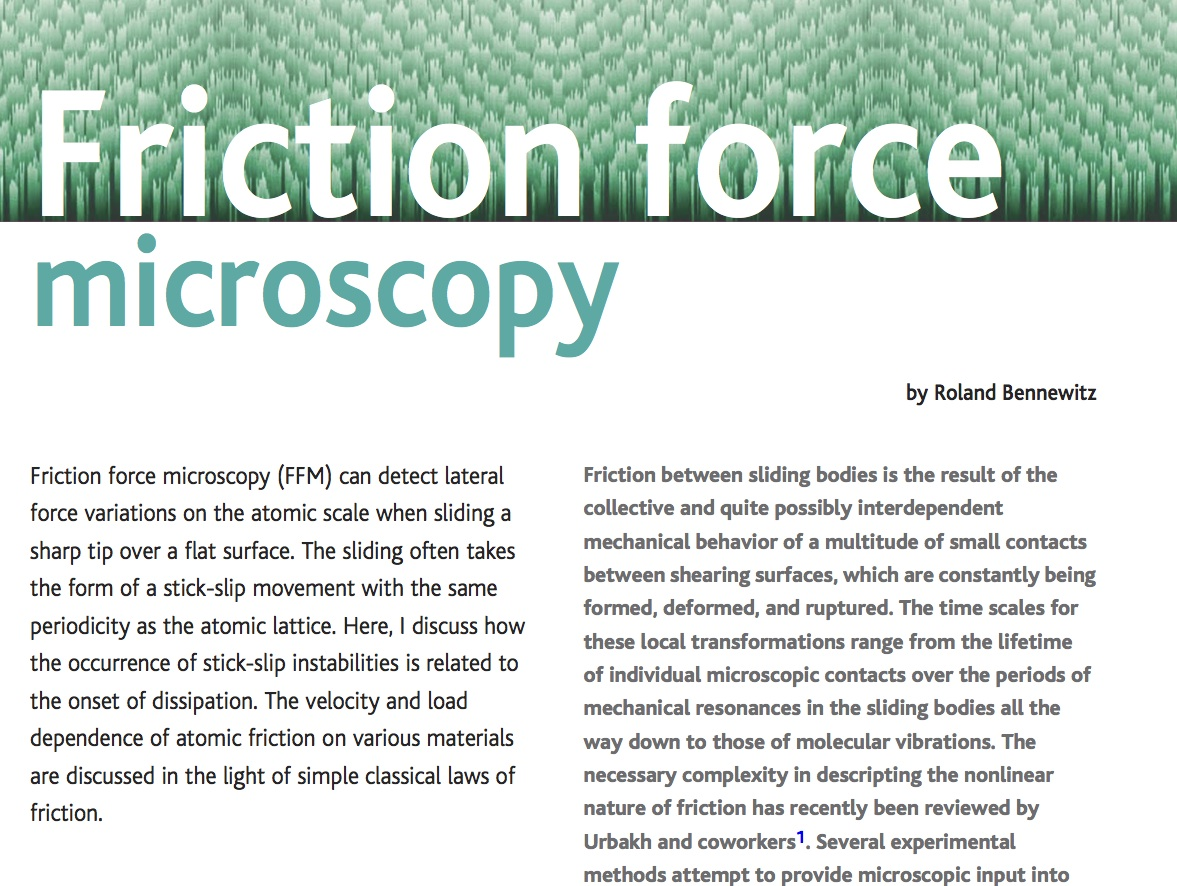Europhys. Lett., 67 (6), p. 907 (2004)

### Static Friction

Some rules:

1. The direction of the static friction force is pointed opposite to the expected motion.
2. The magnitude of $f_s$ changes so that the $F_{net}$ is zero and the object stays in static equilibrium (i.e. not moving)
3. The magnitude of $f_s$ cannot be larger than: $$f_s^\textrm{MAX} = \mu_s n$$ [$\mu_s$ : coefficient of static friction and $n$ : normal force]

1) The direction of the static friction force $\mathbf{f}_s$ is pointed opposite to the expected motion.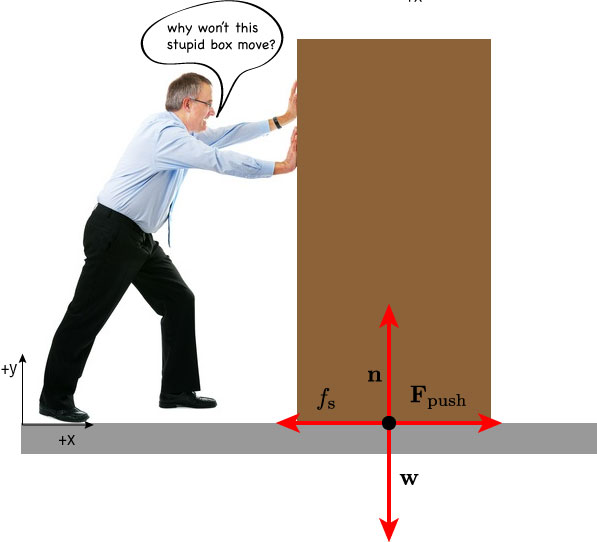2) The magnitude of $f_s$ changes so that the $F_\textrm{net}$ is zero and the object stays in static equilibrium (i.e. not moving)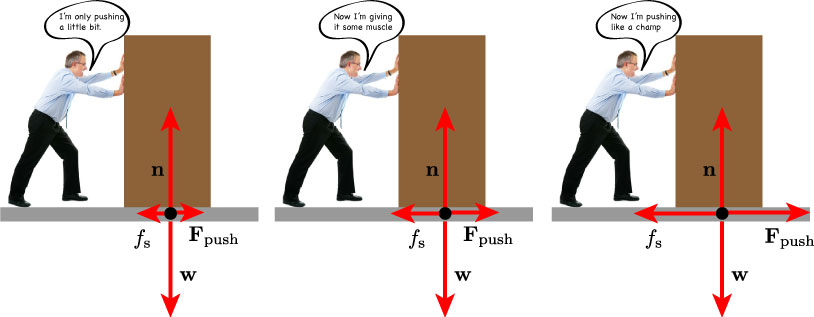3) The magnitude of $f_s$ cannot be larger than: $$f_s^\textrm{MAX} = \mu_s n$$

Eventually, if the $F_\textrm{push}$ is large enough, the $\mu_s$ will no longer be able to cancel the applied force and the box will begin to accelerate in the direction of $F_\textrm{net}$.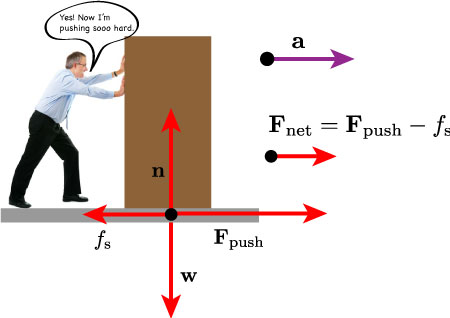I'm pushing in the positive x direction against a box of mass $m$. It doesn't move because of static friction (coefficent is $\mu_s$) between it and the floor...

### Which brings us to kinetic friction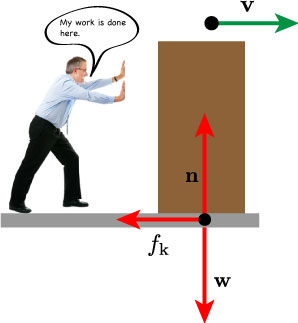Now that the box is in motion, the friction force is replaced by $f_k$ . $$f_k = \mu_k n$$

[$\mu_k$ : coefficient of kinetic friction and $n$ : normal force]

Again, it’s really a microscopic phenomenon, but we can make model about how it behaves. Kinetic friction is proportional to the normal force and doesn’t depend on the velocity with which the object is sliding.

#### Some example coefficients of friction

MaterialsStatic Friction $\mu_s$Kinetic Friction $\mu_k$
Glass on Glass0.940.4
Ice on ice0.10.02
Rubber on Dry Concrete1.00.8
Rubber on Wet Concrete0.70.5
Steel on Ice0.10.05
Teflon on Teflon0.040.04
Wood on Wood0.350.3

A 4000 kg rock is placed on a 15º slope. It stays put. What is the frictional force on the rock?

I push a wooden block across a wood floor at a steady speed of 2.0 m/s. The block has a mass of 10kg. How much force am I exerting on the box? The $\mu_k$ for wood on wood is 0.20.

A downward force $\overrightarrow{F}$ is a applied to the box as shown. How strong does it need to be so that the box doesn't slide? The static friction coefficient is 0.35 between the box and the floor.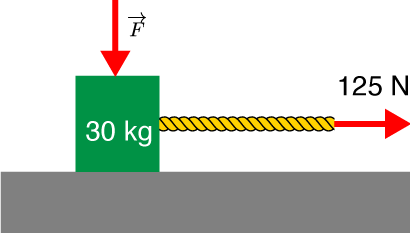### Interacting Objects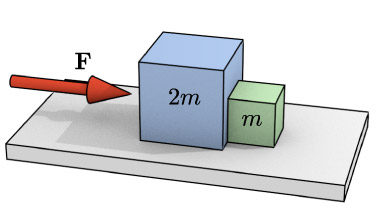A force of magnitude $F$ pushes a block of mass 2m, which in turn pushes a block of mass m, as shown. The blocks are accelerated across a horizontal, frictionless surface. What is the magnitude (in terms of $F$) of the force that the smaller block exerts on the larger block?

#### DragThe drag force $D$, is just as complicated as friction. Again, we have to make some approximations to reality in order to be able to work with drag. In general, drag will be:

1. Opposite to the direction of the velocity vector
2. Increases in magnitude as the objects speed increases.

We also have to assume:

1. the object is not very small or very big
2. the speed is less than a few hundred meters per second
3. the object is moving through the air near the earths surface

## Drag

If the above conditions are met, then we can estimate the drag force by the following:

$$\mathbf{D} = \frac{1}{2}C_D \rho A v^2$$

where,

• $C_D$ is the drag coefficient
• $\rho$ is the density of air
• $A$ is the cross-sectional area of the object
• $v$ is the speed of the object.

#### Terminal Velocity

We know the $F_\textrm{net}$ is proportional to the acceleration of an object. In a vacuum, for an object in free fall, the only force which contributes to $F_\textrm{net}$ is the force of gravity: $\mathbf{F}_G$.

However, in the presence of a medium (air), then D, the drag force must also be accounted for in the calculation of $F_\textrm{net}$.

$$F_\textrm{net} = D - F_G$$

#### Terminal Velocity

Eventually, since Drag is proportional to $v^2$, the two forces will cancel, leading to an $F_\textrm{net} = 0$, and thus no acceleration. This is terminal velocity.

$$D = F_G$$ $$\frac{1}{2} C \rho A v_t^2 - F_G = 0$$ $$v_t = \sqrt{\frac{2F_g}{C \rho A}}$$

How does the size of a hailstone affect its terminal velocity? Do bigger hailstones fall faster than smaller ones? (you can assume uniform density)

$$v_t = \sqrt{\frac{2F_g}{C \rho A}}$$1. Bigger Hailstones fall faster
2. Smaller Hailstones fall faster
3. They all have the same $v_t$

## Forces, in Modern Physics

Property/Interaction Gravitation Weak Electromagnetic Strong
(Electroweak) Fundamental Residual
Acts on: Mass - Energy Flavor Electric charge Color charge Atomic nuclei
Particles experiencing: All Quarks, leptons Electrically charged Quarks, Gluons Hadrons
Particles mediating: Not yet observed
(Graviton hypothesised)
W+ W Z0 γ (photon) Gluons Mesons